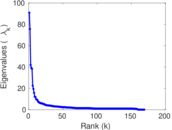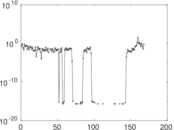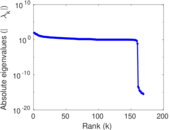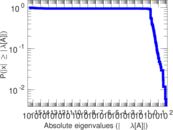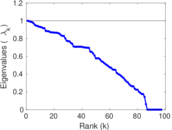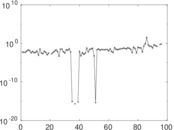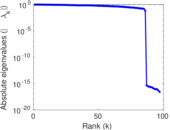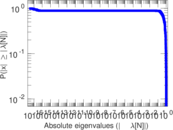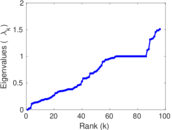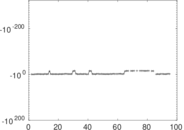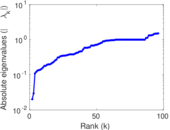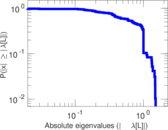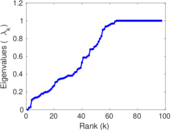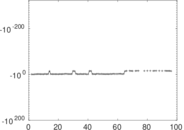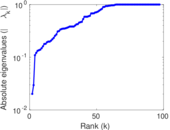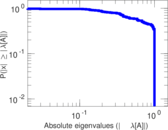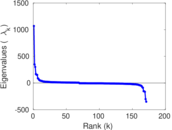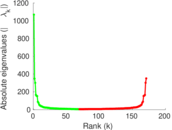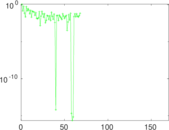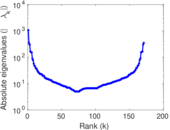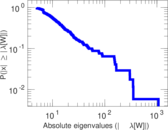# Wiktionary edits (ts)

This is the bipartite edit network of the Tsonga Wiktionary. It contains users and pages from the Tsonga Wiktionary, connected by edit events. Each edge represents an edit. The dataset includes the timestamp of each edit.

 Code `mts` Internal name `edit-tswiktionary` Name Wiktionary edits (ts) Data source http://dumps.wikimedia.org/ AvailabilityDataset is available for download Consistency checkDataset passed all tests Category Authorship network Dataset timestamp 2017-10-20 Node meaning User, article Edge meaning Edit Network formatBipartite, undirected Edge typeUnweighted, multiple edges Temporal dataEdges are annotated with timestamps

## Statistics

 Size n = 1,079 Left size n1 = 171 Right size n2 = 908 Volume m = 2,756 Unique edge count m̿ = 1,582 Wedge count s = 94,523 Claw count z = 7,322,023 Cross count x = 516,151,597 Square count q = 22,952 4-Tour count T4 = 565,180 Maximum degree dmax = 760 Maximum left degree d1max = 760 Maximum right degree d2max = 120 Average degree d = 5.108 43 Average left degree d1 = 16.117 0 Average right degree d2 = 3.035 24 Fill p = 0.010 188 8 Average edge multiplicity m̃ = 1.742 10 Size of LCC N = 796 Diameter δ = 12 50-Percentile effective diameter δ0.5 = 3.269 11 90-Percentile effective diameter δ0.9 = 4.988 21 Median distance δM = 4 Mean distance δm = 3.634 90 Gini coefficient G = 0.736 451 Balanced inequality ratio P = 0.201 379 Left balanced inequality ratio P1 = 0.138 607 Right balanced inequality ratio P2 = 0.272 859 Relative edge distribution entropy Her = 0.817 069 Power law exponent γ = 3.332 07 Tail power law exponent γt = 2.191 00 Tail power law exponent with p γ3 = 2.191 00 p-value p = 0.000 00 Left tail power law exponent with p γ3,1 = 1.731 00 Left p-value p1 = 0.505 000 Right tail power law exponent with p γ3,2 = 2.961 00 Right p-value p2 = 0.000 00 Degree assortativity ρ = −0.229 663 Degree assortativity p-value pρ = 2.215 70 × 10−20 Spectral norm α = 90.903 1 Algebraic connectivity a = 0.020 153 3 Spectral separation |λ1[A] / λ2[A]| = 1.202 69 Controllability C = 752 Relative controllability Cr = 0.701 493

## Plots

### Fruchterman–Reingold graph drawing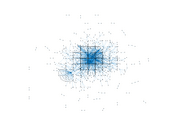### Degree distribution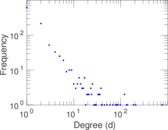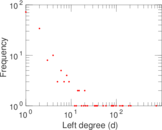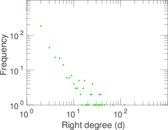### Cumulative degree distribution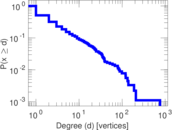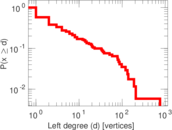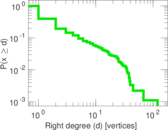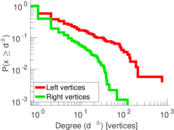### Lorenz curve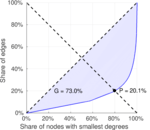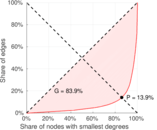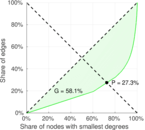### Spectral distribution of the adjacency matrix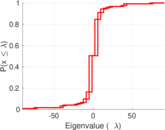### Spectral distribution of the normalized adjacency matrix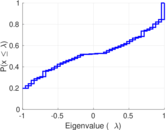### Spectral distribution of the Laplacian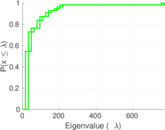### Spectral graph drawing based on the adjacency matrix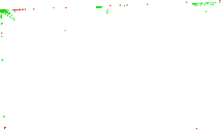### Spectral graph drawing based on the Laplacian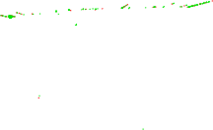### Spectral graph drawing based on the normalized adjacency matrix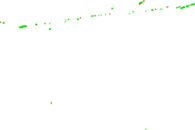### Degree assortativity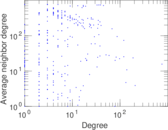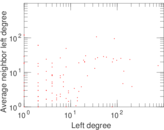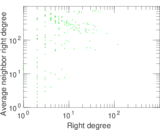### Zipf plot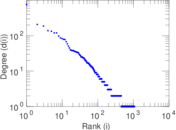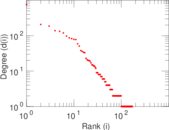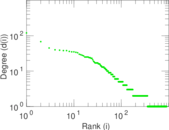### Hop distribution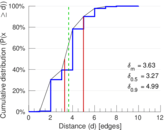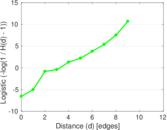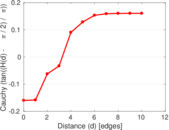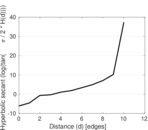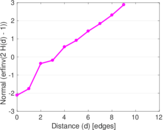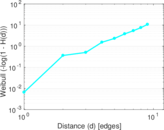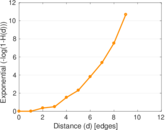### Double Laplacian graph drawing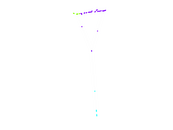### Delaunay graph drawing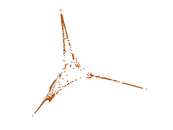### Edge weight/multiplicity distribution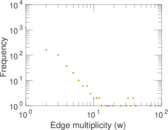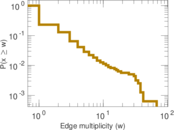### Temporal distribution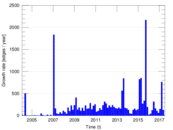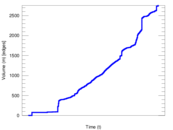### Temporal hop distribution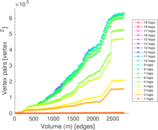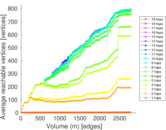### Diameter/density evolution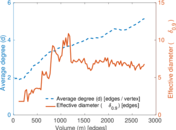### Matrix decompositions plots